# What value resistor will discharge a 2.60 μF capacitor to 20.0% of its initial charge in 2.50 ms?

Part A

What value resistor will discharge a 2.60 μF capacitor to 20.0% of its initial charge in 2.50 ms?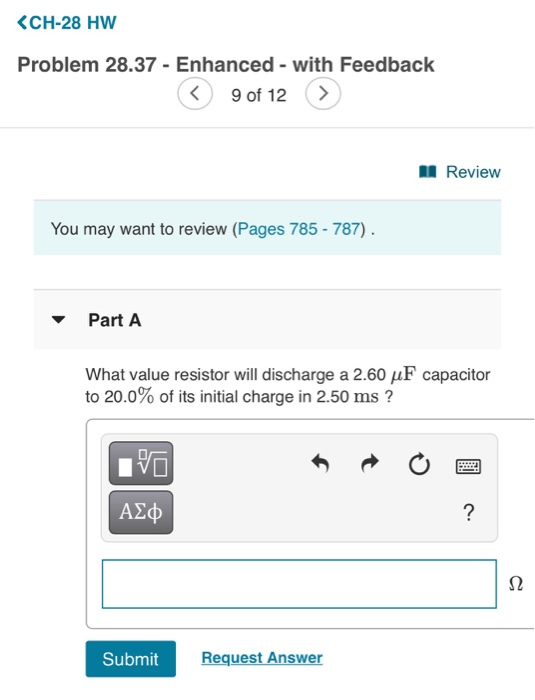SOLUTION :

We use the following formula :

Q = Q0 e^(- t / R C)

=> Q / Q0 = e^ ( - t / RC)

where,

Q / Q0 = 0.20

t = time taken in secs for discharge to 0.20 Q0 = 2.50 ms = 2.50^10^(- 3) sec.

R = Resistance  in Ωs  to be determined .

C = Capacitor capacitance = 2.60 µF= 2.60*10^(-6) F .

Hence,

0.20 =  e^(- 2.50*10^(-3) / (R * 2.60*10^(-6))

=> 0.20 = e^(- 961.53846 / R)

Taking natural logarithm :

=> ln(0.2) = - 961.53846 / R

=> R = - 961.53846 / ln(0.2)

=> R = 597.44 Ω   approx.

=> Resistor of 597.44  Ω approx. (ANSWER).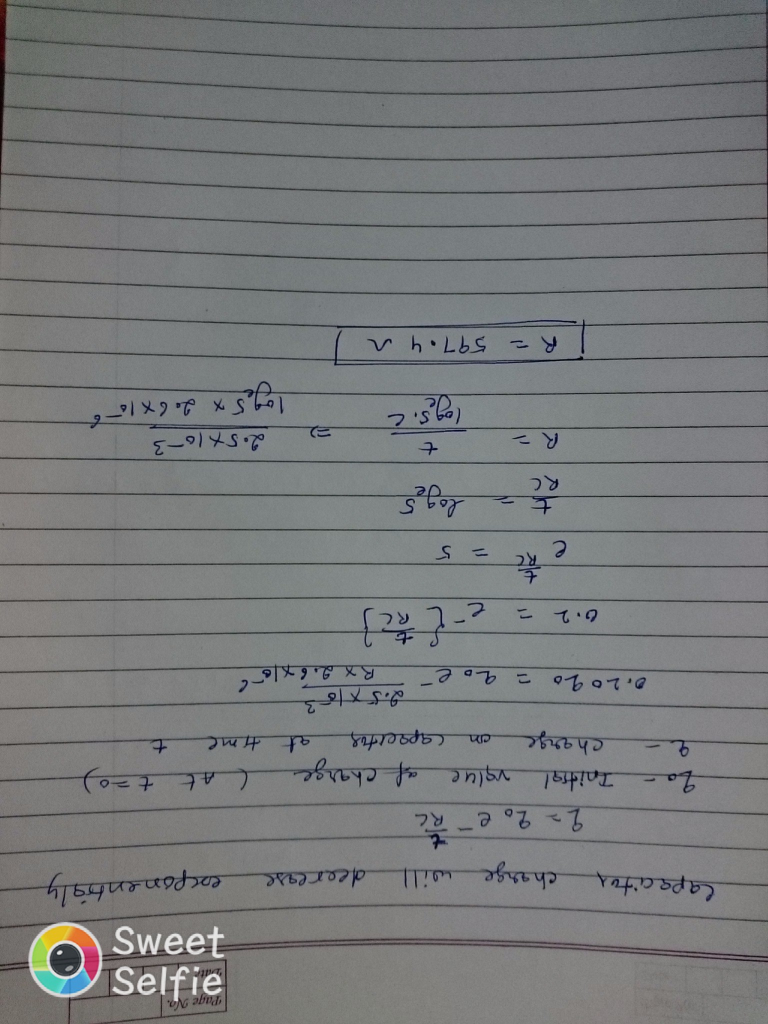#### Earn Coin

Coins can be redeemed for fabulous gifts.

Similar Homework Help Questions
• ### What value resistor will discharge a 2.60 μF capacitor to 20.0 % of its initial charge in 2.50 ms

Part AWhat value resistor will discharge a 2.60 μF capacitor to 20.0 % of its initial charge in 2.50 ms?

• ### Part A What value resistor will discharge a 2.50 uF capacitor to 20.0% of its initial...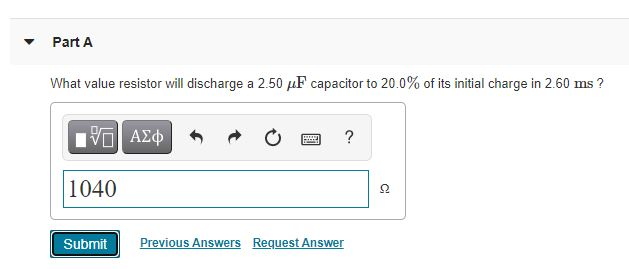Part A What value resistor will discharge a 2.50 uF capacitor to 20.0% of its initial charge in 2.60 ms? IVO AO ? 1040 2 Submit Previous Answers Request Answer

• ### What value resistor will discharge a 2.40 μF capacitor to 20.0% of its initial charge in...

What value resistor will discharge a 2.40 μF capacitor to 20.0% of its initial charge in 3.00 ms ?

• ### What value resistor will discharge a 2.1 μF capacitor to 20 % of its initial charge...

What value resistor will discharge a 2.1 μF capacitor to 20 % of its initial charge in 2.4 ms ?

• ### What value resistor will discharge a 3.0 μF capacitor to 10 % of its initial charge...

What value resistor will discharge a 3.0 μF capacitor to 10 % of its initial charge in 2.1 ms ?

• ### What value resistor will discharge a 2.1 μF capacitor to 20 % of its initial charge...

What value resistor will discharge a 2.1 μF capacitor to 20 % of its initial charge in 2.8 ms ?

• ### What value resistor will discharge a 3.00microfarads capacitor to 20.0% of its initial charge in...

What value resistor will discharge a 3.00microfarads capacitor to 20.0% of its initial charge in 2.10ms ?

• ### If you want to discharge a 8.00 μF capacitor to 55.0% of its initial charge in...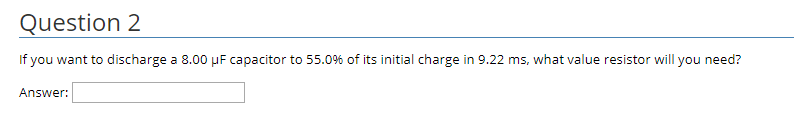If you want to discharge a 8.00 μF capacitor to 55.0% of its initial charge in 9.22 ms, what value resistor will you need? Question 2 If you want to discharge a 8.00 μ F capacitor to 55.0% of its initial charge in 9.22 ms, what value resistor will you need? Answer:

• ### What value resistor will discharge a 2.80μF capacitor to 10.0% of its initial charge in 3.00ms ? Part A What value r...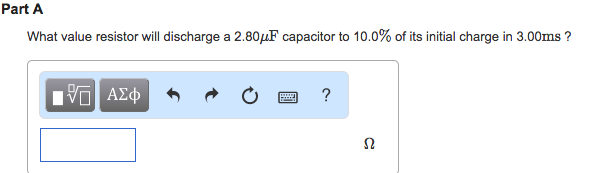What value resistor will discharge a 2.80μF capacitor to 10.0% of its initial charge in 3.00ms ?Part A What value resistor will discharge a 2.80 MuF capacitor to 10.0% of its initial charge in 3.00ms?

• ### resistor value

What value resistor will discharge a 2.40 uF capacitor to 20.0% of its initial charge in 2.60 ms?